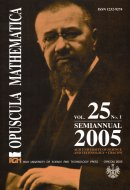Opuscula Math. 25, no. 1 (2005), 5-24

Opuscula Mathematica

# Solution of the Stieltjes truncated matrix moment problem

Igor M. Tkachenko

Abstract. The truncated Stieltjes matrix moment problem consisting in the description of all matrix distributions $$\boldsymbol{\sigma}(t)$$ on $$[0,\infty)$$ with given first $$2n+1$$ power moments $$(\mathbf{C}_j)_{n=0}^j$$ is solved using known results on the corresponding Hamburger problem for which $$\boldsymbol{\sigma}(t)$$ are defined on $$(-\infty,\infty)$$. The criterion of solvability of the Stieltjes problem is given and all its solutions in the non-degenerate case are described by selection of the appropriate solutions among those of the Hamburger problem for the same set of moments. The results on extensions of non-negative operators are used and a purely algebraic algorithm for the solution of both Hamburger and Stieltjes problems is proposed.

Keywords: Stieltjes power moments, canonical solutions, Nevanlinna's formula.

Mathematics Subject Classification: 30E05, 30E10.

Full text (pdf)

• I. I. Mechnikov Odessa National University, Department of Theoretical Physics, 65026 Odessa, Ukraine
• Igor M. Tkachenko
• Polytechnic University of Valencia, Department of Applied Mathematics, 46022 Valencia, Spain